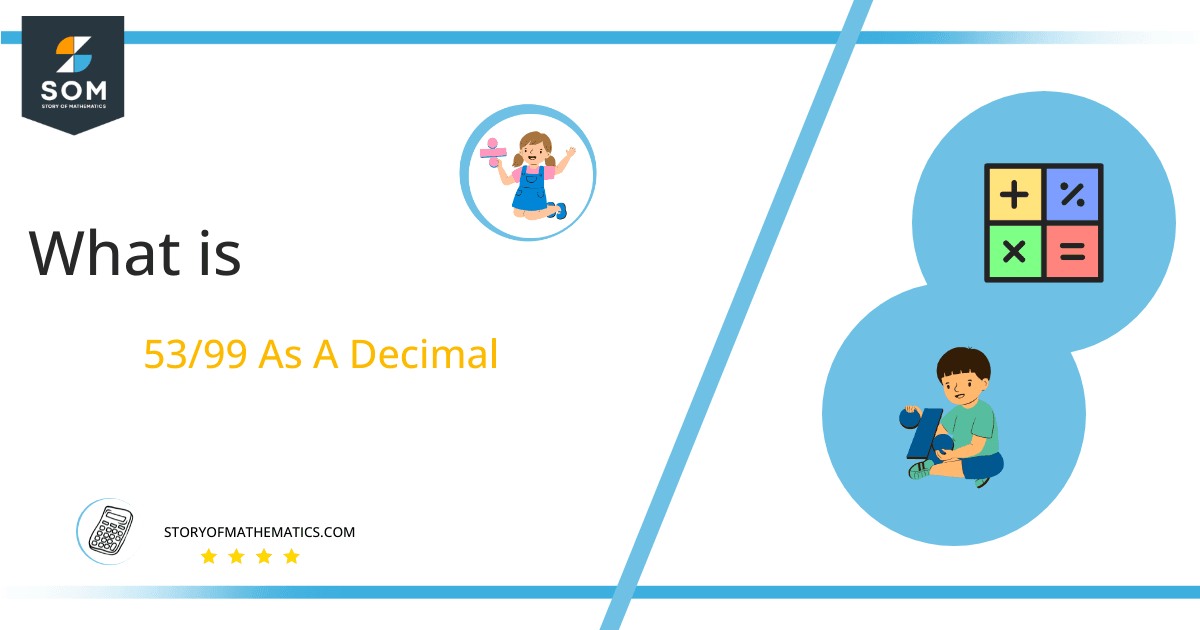# What Is 53/99 as a Decimal + Solution With Free Steps

The fraction 53/99 as a decimal is equal to 0.535.

Fractional numbers are a culmination of two numbers being divided by each other. The upper number is the numerator value which is the dividend in the long division and the lower value is the denominator which is the divisor in the division process.Here, we are more interested in the division types that result in a Decimal value, as this can be expressed as a Fraction. We see fractions as a way of showing two numbers having the operation of Division between them that result in a value that lies between two Integers.

Now, we introduce the method used to solve said fraction to decimal conversion, called Long Division, which we will discuss in detail moving forward. So, let’s go through the Solution of fraction 53/99.

## Solution

First, we convert the fraction components, i.e., the numerator and the denominator, and transform them into the division constituents, i.e., the Dividend and the Divisor, respectively.

This can be done as follows:

Dividend = 53

Divisor = 99

Now, we introduce the most important quantity in our division process: the Quotient. The value represents the Solution to our division and can be expressed as having the following relationship with the Division constituents:

Quotient = Dividend $\div$ Divisor = 53 $\div$ 99

This is when we go through the Long Division solution to our problem. Given is the long division process in Figure 1:Figure 1

## 53/99 Long Division Method

We start solving a problem using the Long Division Method by first taking apart the division’s components and comparing them. As we have 53 and 99, we can see how 53 is Smaller than 99, and to solve this division, we require that 53 be Bigger than 99.

This is done by multiplying the dividend by 10 and checking whether it is bigger than the divisor or not. If so, we calculate the Multiple of the divisor closest to the dividend and subtract it from the Dividend. This produces the Remainder, which we then use as the dividend later.

Now, we begin solving for our dividend 53, which after getting multiplied by 10 becomes 530.

We take this 530 and divide it by 99; this can be done as follows:

530 $\div$ 99 $\approx$ 5

Where:

99 x 5 = 495

This will lead to the generation of a Remainder equal to 530 – 495 = 35. Now this means we have to repeat the process by Converting the 35 into 350 and solving for that:

350 $\div$ 99 $\approx$ 3

Where:

99 x 3 = 297

This, therefore, produces another Remainder which is equal to 350 – 297 = 53. Now we must solve this problem to Third Decimal Place for accuracy, so we repeat the process with dividend 530.

530 $\div$ 99 $\approx$ 5

Where:

99 x 5 = 495

Finally, we have a Quotient generated after combining the three pieces of it as 0.535, with a Remainder equal to 35.Images/mathematical drawings are created with GeoGebra.